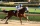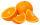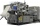# Fraction calculator

The calculator performs basic and advanced operations with fractions, expressions with fractions combined with integers, decimals, and mixed numbers. It also shows detailed step-by-step information about the fraction calculation procedure. Solve problems with two, three, or more fractions and numbers in one expression.

## Result:

### 11/5 - 1/3 = 13/15 ≅ 0.8666667

Spelled result in words is thirteen fifteenths.

### How do you solve fractions step by step?

1. Conversion a mixed number 1 1/5 to a improper fraction: 1 1/5 = 1 1/5 = 1 · 5 + 1/5 = 5 + 1/5 = 6/5

To find new numerator:
a) Multiply the whole number 1 by the denominator 5. Whole number 1 equally 1 * 5/5 = 5/5
b) Add the answer from previous step 5 to the numerator 1. New numerator is 5 + 1 = 6
c) Write a previous answer (new numerator 6) over the denominator 5.

One and one fifth is six fifths
2. Subtract: 6/5 - 1/3 = 6 · 3/5 · 3 - 1 · 5/3 · 5 = 18/15 - 5/15 = 18 - 5/15 = 13/15
For adding, subtracting, and comparing fractions, it is suitable to adjust both fractions to a common (equal, identical) denominator. The common denominator you can calculate as the least common multiple of both denominators - LCM(5, 3) = 15. In practice, it is enough to find the common denominator (not necessarily the lowest) by multiplying the denominators: 5 × 3 = 15. In the next intermediate step, the fraction result cannot be further simplified by canceling.
In words - six fifths minus one third = thirteen fifteenths.

#### Rules for expressions with fractions:

Fractions - use the slash “/” between the numerator and denominator, i.e., for five-hundredths, enter 5/100. If you are using mixed numbers, be sure to leave a single space between the whole and fraction part.
The slash separates the numerator (number above a fraction line) and denominator (number below).

Mixed numerals (mixed fractions or mixed numbers) write as non-zero integer separated by one space and fraction i.e., 1 2/3 (having the same sign). An example of a negative mixed fraction: -5 1/2.
Because slash is both signs for fraction line and division, we recommended use colon (:) as the operator of division fractions i.e., 1/2 : 3.

Decimals (decimal numbers) enter with a decimal point . and they are automatically converted to fractions - i.e. 1.45.

The colon : and slash / is the symbol of division. Can be used to divide mixed numbers 1 2/3 : 4 3/8 or can be used for write complex fractions i.e. 1/2 : 1/3.
An asterisk * or × is the symbol for multiplication.
Plus + is addition, minus sign - is subtraction and ()[] is mathematical parentheses.
The exponentiation/power symbol is ^ - for example: (7/8-4/5)^2 = (7/8-4/5)2

#### Examples:

subtracting fractions: 2/3 - 1/2
multiplying fractions: 7/8 * 3/9
dividing Fractions: 1/2 : 3/4
exponentiation of fraction: 3/5^3
fractional exponents: 16 ^ 1/2
adding fractions and mixed numbers: 8/5 + 6 2/7
dividing integer and fraction: 5 ÷ 1/2
complex fractions: 5/8 : 2 2/3
decimal to fraction: 0.625
Fraction to Decimal: 1/4
Fraction to Percent: 1/8 %
comparing fractions: 1/4 2/3
multiplying a fraction by a whole number: 6 * 3/4
square root of a fraction: sqrt(1/16)
reducing or simplifying the fraction (simplification) - dividing the numerator and denominator of a fraction by the same non-zero number - equivalent fraction: 4/22
expression with brackets: 1/3 * (1/2 - 3 3/8)
compound fraction: 3/4 of 5/7
fractions multiple: 2/3 of 3/5
divide to find the quotient: 3/5 ÷ 2/3

The calculator follows well-known rules for order of operations. The most common mnemonics for remembering this order of operations are:
PEMDAS - Parentheses, Exponents, Multiplication, Division, Addition, Subtraction.
BEDMAS - Brackets, Exponents, Division, Multiplication, Addition, Subtraction
BODMAS - Brackets, Of or Order, Division, Multiplication, Addition, Subtraction.
GEMDAS - Grouping Symbols - brackets (){}, Exponents, Multiplication, Division, Addition, Subtraction.
Be careful, always do multiplication and division before addition and subtraction. Some operators (+ and -) and (* and /) has the same priority and then must evaluate from left to right.

## Fractions in word problems:

• SchoolThere are 150 pupils in grade 5 . 2/3 of them are female. By what fractions are the males?
• Wood 11Father has 12 1/5 meters long wood. Then I cut the wood into two pieces. One part is 7 3/5 meters long. Calculate the length of the other wood?
• Mixed numbersRewrite mixed numbers, so the fractions have the same denominator: 5 1/5 - 2 2/3
• HusseinHussein owns 450000 crowns (local currency). He spent at the bookstore 2 over 9 to buy some books and tales. He paid 3 over 5 of his money to buy his math book. a. Calculate the remaining amount of money with Hussein? b. Hussein lost 3 over 4 of the remai
• EricaErica bought 3 1/2 yards of fabric. If she uses 2/3 of the fabric, how much will she have left?
• Simplify 3Simplify mixed numerals expression: 8 1/4- 3 2/5 - (2 1/3 - 1/4) Show your solution.
• Square metal sheetWe cut out four squares of 300 mm side from a square sheet metal plate with a side of 0,7 m. Express the fraction and the percentage of waste from the square metal sheet.Sadie practiced her spelling words for 3/4 of an hour, and Max practiced his spelling words for 5/12 of an hour. In the simplest form, how much longer did Sadie practice than Max?Of 100 types of animals, 9/100 were discovered in ancient times, and 2/100 were discovered in the Middle Ages. Another 3/10 were discovered in the 1800s. What fraction of the 100 types of animals was discovered after the 1800s? Explain.A number, X, is subtracted from 8 1/4. The result is 12 3/5. What is the value of X?In bucket of 87 oranges 1/3 are spoiled ones and the remaining are eaten by the member of a family. How many oranges are eaten by the member of family?Erika’s career consists of filing, typing and answering phones. She spends 1/6 of her time filing and 5/8 of her time typing. What fraction of her time does she spend answering phone calls?Mr. Green washed 1/4 of his laundry. His son washed 3/7 of it. Who washed most of the laundry? How much of the laundry still needs to be washed?